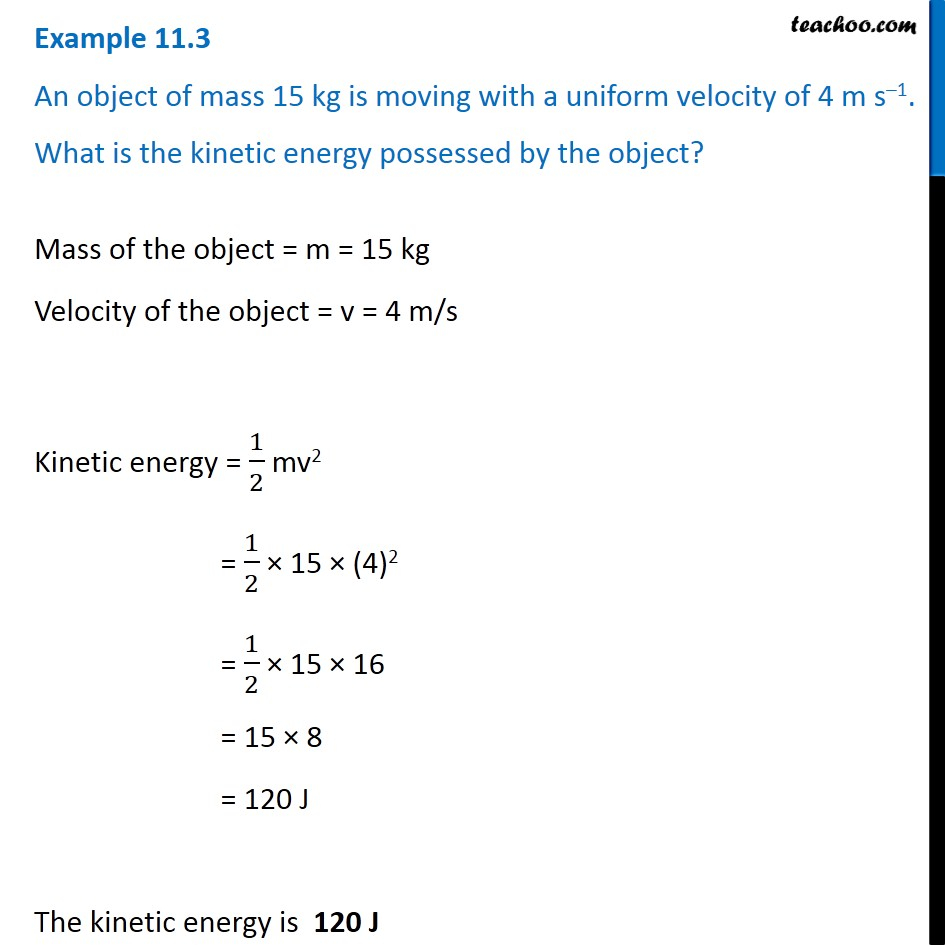Examples from NCERT Book

Class 9
Chapter 11 Class 9 - Work and EnergyLearn in your speed, with individual attention - Teachoo Maths 1-on-1 Class

### Transcript

Example 11.3 An object of mass 15 kg is moving with a uniform velocity of 4 m s–1. What is the kinetic energy possessed by the object? Mass of the object = m = 15 kg Velocity of the object = v = 4 m/s Kinetic energy = 1/2 mv2 = 1/2 × 15 × (4)2 = 1/2 × 15 × 16 = 15 × 8 = 120 J The kinetic energy is 120 J